# Pytorch实现Actor Critic Baseline (A2C, Cliff Walking PlayGround)2020年8月21日07:21:21

6637字阅读22分7秒

## Actor Critic Baseline的介绍

Actor Critic是Policy Gradient算法. 他的想法就是希望可以找到一个函数, 来近似Policy, 给这个函数state, 返回的是每一个动作的action. 一般我们会称这个policy为Pi.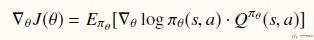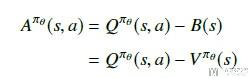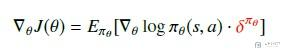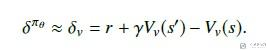### Actor Critic Baseline算法流程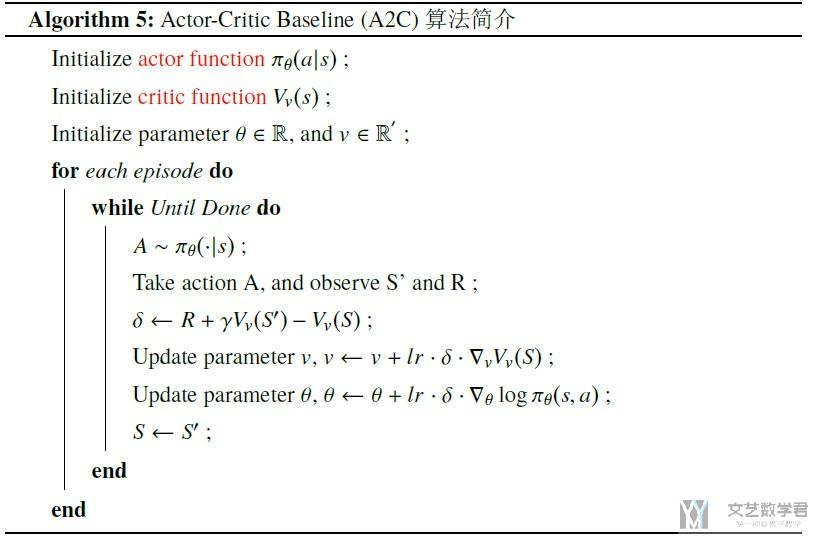• 为了更新critic function, 我们设置loss function为(V-Gt)^2, 求导后与上面的式子相同.
• 为了更新actor function, 我们设置loss function为-log_prob * (Gt - V), 这里我们增加负号, 于是可以使用梯度下降来进行参数更新.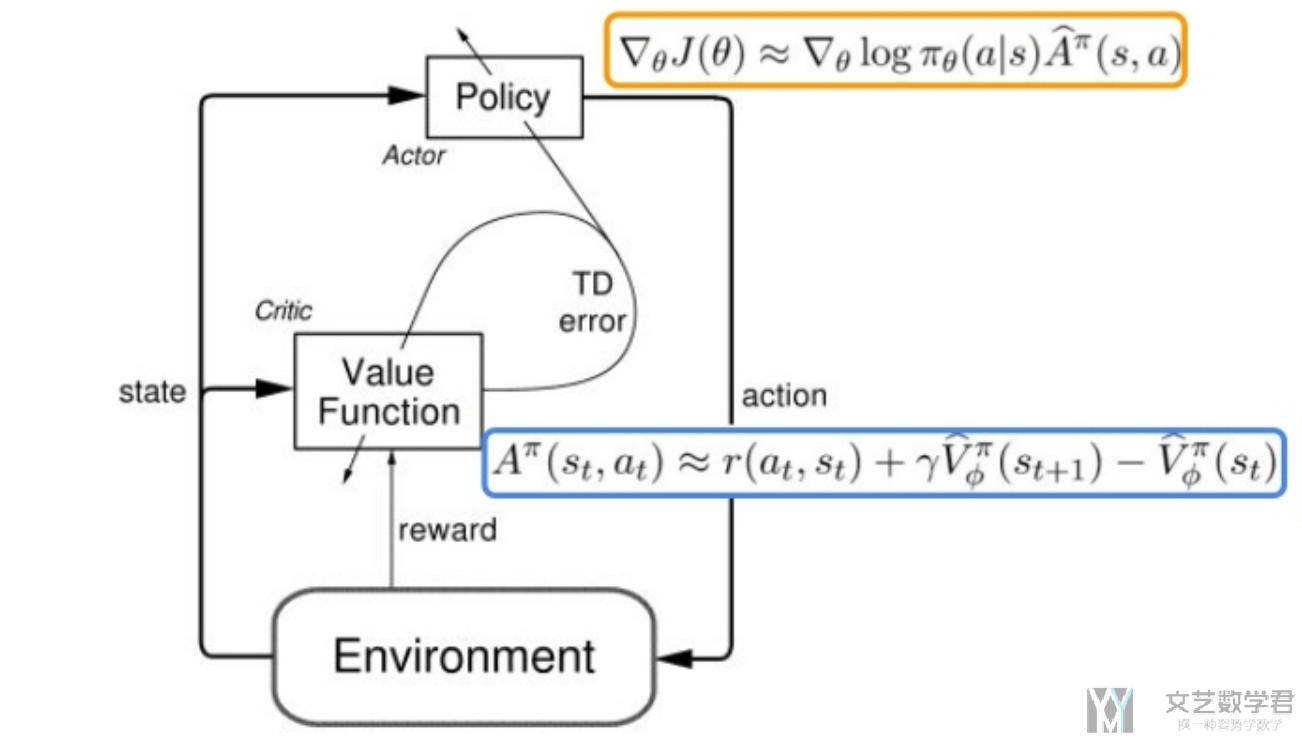## Pytorch实现Actor Critic Baseline (A2C)

### 初始化环境

1. env = CliffWalkingEnv()

1. def get_screen(state):
2.     """这里我们就用state来作为例子, 不直接使用截图了, 将编号转换为one-hot向量, 共48维
3.     """
4.     y_state = torch.Tensor([[state]]).long()
5.     y_onehot = torch.FloatTensor(1, 48) # 产生位置
6.     # In your for loop
7.     y_onehot.zero_() # 全部使用0进行填充
8.     y_onehot.scatter_(1, y_state, 1) # 返回one-hot
9.     return y_onehot

1. get_screen(1)
2. """
3. tensor([[0., 1., 0., 0., 0., 0., 0., 0., 0., 0., 0., 0., 0., 0., 0., 0., 0., 0.,
4.          0., 0., 0., 0., 0., 0., 0., 0., 0., 0., 0., 0., 0., 0., 0., 0., 0., 0.,
5.          0., 0., 0., 0., 0., 0., 0., 0., 0., 0., 0., 0.]])
6. """

### 定义Actor Critic的模型

• Actor模型的输入是state, 输出是每一个action的概率.
• Critic模型的输入是state, 输入是这个state对应的value.

1. class ActorCriticModel(nn.Module):
2.     def __init__(self):
3.         super(ActorCriticModel, self).__init__()
4.         self.fc1 = nn.Linear(48, 24)
5.         self.fc2 = nn.Linear(24, 12)
6.         self.action = nn.Linear(12, 4)
7.         self.value = nn.Linear(12, 1)
8.     def forward(self, x):
9.         x = F.relu(self.fc1(x))
10.         x = F.relu(self.fc2(x))
11.         action_probs = F.softmax(self.action(x), dim=-1)
12.         state_values = self.value(x)
13.         return action_probs, state_values

• Actor模型的结构是, 48->24->12->4;
• Critic模型的结构是, 48->24->12->1;

1. ac = ActorCriticModel()
2. action_probs, state_values = ac(get_screen(1).squeeze(0))
3. print(action_probs)
4. print(state_values)
5. """
6. tensor([0.2140, 0.3271, 0.2130, 0.2459], grad_fn=<SoftmaxBackward>)
8. """

### 模型的训练

• 当前state的累计收益(Gt, 这个是实际计算的);
• 对当前state收益的估计(V值);
• 当前state采取一个action的概率的log值, 即对应下面代码中的log_probs;

• critic loss = (V-Gt)^2, 也就是希望我们对状态state的价值估计与其实际价值相似.
• actor loss = -log_prob * (Gt - V), 我们称作Gt-V为advantage function.

1. def trainIters(env, ActorCriticModel, num_episodes, gamma = 0.9):
2.     optimizer = torch.optim.Adam(ActorCriticModel.parameters(), 0.03) # 注意学习率的大小
3.     scheduler = torch.optim.lr_scheduler.StepLR(optimizer, step_size=10, gamma=0.9)
4.     # 记录reward和总长度的变化
5.     stats = plotting.EpisodeStats(
6.         episode_lengths=np.zeros(num_episodes+1),
7.         episode_rewards=np.zeros(num_episodes+1))
8.     for i_episode in range(1, num_episodes+1):
9.         # 开始一轮游戏
10.         state = env.reset() # 环境重置
11.         state = get_screen(state) # 将state转换为one-hot的tensor, 用作网络的输入.
12.         log_probs = []
13.         rewards = []
14.         state_values = []
15.         for t in itertools.count():
16.             action_probs, state_value = ActorCriticModel(state.squeeze(0)) # 返回当前state下不同action的概率
17.             action = torch.multinomial(action_probs, 1).item() # 选取一个action
18.             log_prob = torch.log(action_probs[action])
19.             next_state, reward, done, _ = env.step(action) # 获得下一个状态
20.             # 计算统计数据
21.             stats.episode_rewards[i_episode] += reward # 计算累计奖励
22.             stats.episode_lengths[i_episode] = t # 查看每一轮的时间
23.             # 将值转换为tensor
24.             reward = torch.tensor([reward], device=device)
25.             next_state_tensor = get_screen(next_state)
26.             # 将信息存入List
27.             log_probs.append(log_prob.view(-1))
28.             rewards.append(reward)
29.             state_values.append(state_value)
30.             # 状态更新
31.             state = next_state_tensor
32.             if done: # 当一轮结束之后, 开始更新
33.                 returns = []
34.                 Gt = 0
35.                 pw = 0
36.                 # print(rewards)
37.                 for reward in rewards[::-1]:
38.                     Gt = Gt + (gamma ** pw) * reward # 写成Gt += (gamma ** pw) * reward, 最后returns里东西都是一样的
39.                     # print(Gt)
40.                     pw += 1
41.                     returns.append(Gt)
42.                 returns = returns[::-1]
43.                 returns = torch.cat(returns)
44.                 returns = (returns - returns.mean()) / (returns.std() + 1e-9)
45.                 # print(returns)
46.                 log_probs = torch.cat(log_probs)
47.                 state_values = torch.cat(state_values)
48.                 # print(returns)
49.                 # print(log_probs)
50.                 # print(state_values)
51.                 advantage = returns.detach() - state_values
52.                 critic_loss = F.smooth_l1_loss(state_values, returns.detach())
53.                 actor_loss = (-log_probs * advantage.detach()).mean()
54.                 loss = critic_loss + actor_loss
55.                 # 更新critic
57.                 loss.backward()
58.                 optimizer.step()
59.                 print('Episode: {}, total steps: {}'.format(i_episode, t))
60.                 if t>20:
61.                     scheduler.step()
62.                 break
63.     return stats

• 对于每一个episode, 我们都进行模型, 使用actor生成动作, 并使用critic得到对state的评价, 将其分别存入数组中.
• 当一个episode结束后, 我们根据返回的reward, 分别计算每一个state的累计reward. 也就是上面`for reward in reward[::-1]`部分的代码. 接着就是计算两个的loss, 并进行反向传播, 更新网络参数.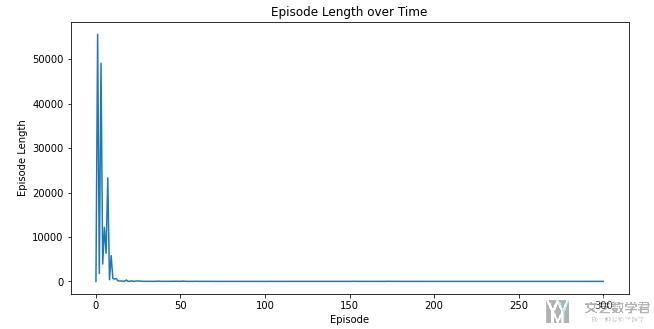### 一些注意点

1. returns = (returns - returns.mean()) / (returns.std() + 1e-9)

1. Gt += (gamma ** pw) * reward,

1. Gt = Gt + (gamma ** pw) * reward

• 微信公众号
• 关注微信公众号
•• QQ群
• 我们的QQ群号
•• 本文由 发表于 2020年8月21日07:21:21
• 转载请务必保留本文链接：https://mathpretty.com/12665.html